Get instant live expert help with Excel or Google Sheets“My Excelchat expert helped me in less than 20 minutes, saving me what would have been 5 hours of work!”

#### Post your problem and you’ll get expert help in seconds.

Your message must be at least 40 characters
Our professional experts are available now. Your privacy is guaranteed.

# How to Round to the Nearest 10 in Excel

Excel is one of the best tools to make complicated works easier with the help of simple formulas. It also helps us to round off complicated numbers with multiple decimals. Let’s find out how.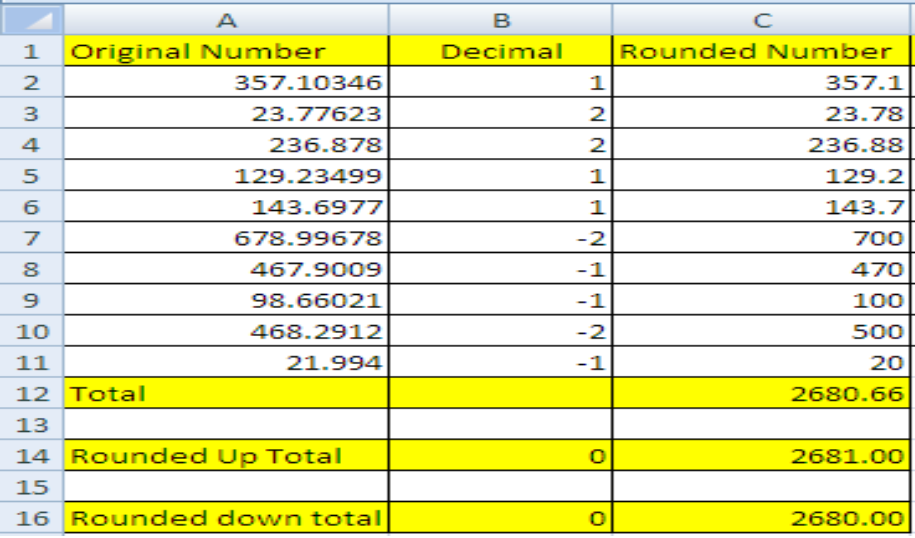Figure 1

Formula: `=Round(number,number_digits)` or `=Round(A2,B2)`

• Column A has the numbers that need to be rounded off and Column B has the number of decimals up to which we want to round off the original number.
• Enter the formula `=Round(A2,B2)` in C2.
• Copy cell C2, select column C and select the option of “pasting formulas” to apply the formula in every cell of C.
• Excel will round off the original number when the number of decimals to be rounded is placed in column B.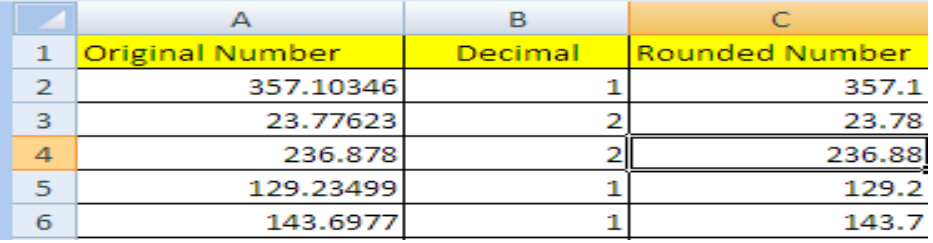Figure 2

• Digits before the decimal point are integers and digits after the decimal point are decimals.
• To round up to the nearest 10 in decimals, we’ll write 1 in decimal column.
• We’ll write 2 in decimal column to round up to nearest 100 in decimals.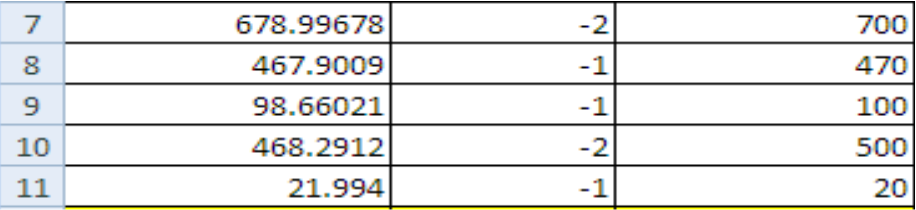Figure 3

• To round up the integer to the nearest 10, write -1 in the B cell next to original number.
• Write -2 to round up the integer up to nearest 100.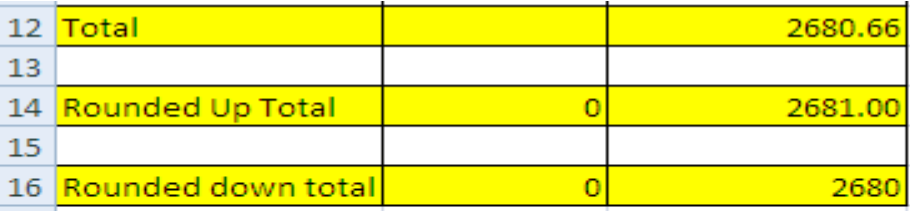Figure 4

Formulas:` =RoundUp(number,number_digits)`

`=RoundDown(number,number_digits)`

• Total of the rounded numbers is 2680.66
• Put 0 in the next cell of the number to remove decimals from the total.
• To take the upper value. We can write formula `=RoundUp(number,number_digits)`
• For the lower value, write `=RoundDown(number,number_digits)`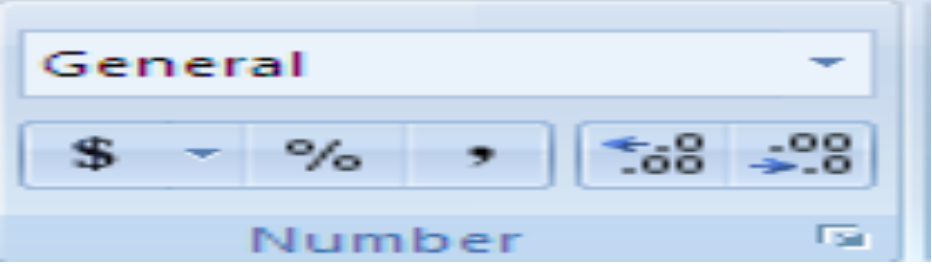Figure 5

Another way to increase or decrease decimals or to round off the original number is to simply click the option of increase/decrease decimal in the lower right corner of the “Number Section” in the toolbar.

Most of the time, the problem you will need to solve will be more complex than a simple application of a formula or function. If you want to save hours of research and frustration, try our live Excelchat service! Our Excel Experts are available 24/7 to answer any Excel question you may have. We guarantee a connection within 30 seconds and a customized solution within 20 minutes.